## 5. A sample of an unknown metal has a mass of 120.4 g. As the sample cools from 90.5°C to 25.7°C, it releases 7020. J of energy. What is the

Question

5. A sample of an unknown metal has a mass of 120.4 g. As the sample cools from 90.5°C to 25.7°C, it releases 7020. J of energy. What is the specific heat of the sample? Identify the metal among those in the data table below. Finally, is this endothermic or exothermic?

in progress 0
3 months 2021-08-15T05:23:17+00:00 1 Answers 3 views 0

1. Answer: The specific heat capacity of the sample is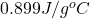and as heat is released in this reaction so it is exothermic in nature.

Explanation:

Given: Mass = 120.4 g

Heat energy released = -7020 J

Initial temperature =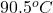Final temperature =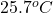Formula used is as follows.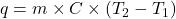where,

q = heat energy

m = mass of substance

C = specific heat capacity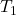= initial temperature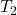= final temperature

Substitute the values into above formula as follows.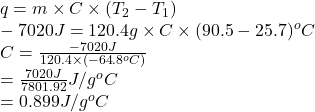When heat is released in a process or reaction then it means it is exothermic in nature.

Thus, we can conclude that the specific heat capacity of the sample isand as heat is released in this reaction so it is exothermic in nature.